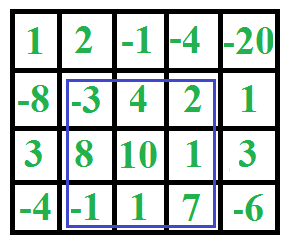# C Program for Maximum sum rectangle in a 2D matrix | DP-27

Given a 2D array, find the maximum sum subarray in it. For example, in the following 2D array, the maximum sum subarray is highlighted with blue rectangle and sum of this subarray is 29.This problem is mainly an extension of Largest Sum Contiguous Subarray for 1D array.

## C

 `// Program to find maximum sum subarray in a given 2D array ` `#include ` `#include ` `#include ` `#define ROW 4 ` `#define COL 5 ` ` `  `// Implementation of Kadane's algorithm for 1D array. The function ` `// returns the maximum sum and stores starting and ending indexes of the ` `// maximum sum subarray at addresses pointed by start and finish pointers ` `// respectively. ` `int` `kadane(``int``* arr, ``int``* start, ``int``* finish, ``int` `n) ` `{ ` `    ``// initialize sum, maxSum and ` `    ``int` `sum = 0, maxSum = INT_MIN, i; ` ` `  `    ``// Just some initial value to check for all negative values case ` `    ``*finish = -1; ` ` `  `    ``// local variable ` `    ``int` `local_start = 0; ` ` `  `    ``for` `(i = 0; i < n; ++i) { ` `        ``sum += arr[i]; ` `        ``if` `(sum < 0) { ` `            ``sum = 0; ` `            ``local_start = i + 1; ` `        ``} ` `        ``else` `if` `(sum > maxSum) { ` `            ``maxSum = sum; ` `            ``*start = local_start; ` `            ``*finish = i; ` `        ``} ` `    ``} ` ` `  `    ``// There is at-least one non-negative number ` `    ``if` `(*finish != -1) ` `        ``return` `maxSum; ` ` `  `    ``// Special Case: When all numbers in arr[] are negative ` `    ``maxSum = arr; ` `    ``*start = *finish = 0; ` ` `  `    ``// Find the maximum element in array ` `    ``for` `(i = 1; i < n; i++) { ` `        ``if` `(arr[i] > maxSum) { ` `            ``maxSum = arr[i]; ` `            ``*start = *finish = i; ` `        ``} ` `    ``} ` `    ``return` `maxSum; ` `} ` ` `  `// The main function that finds maximum sum rectangle in M[][] ` `void` `findMaxSum(``int` `M[][COL]) ` `{ ` `    ``// Variables to store the final output ` `    ``int` `maxSum = INT_MIN, finalLeft, finalRight, finalTop, finalBottom; ` ` `  `    ``int` `left, right, i; ` `    ``int` `temp[ROW], sum, start, finish; ` ` `  `    ``// Set the left column ` `    ``for` `(left = 0; left < COL; ++left) { ` `        ``// Initialize all elements of temp as 0 ` `        ``memset``(temp, 0, ``sizeof``(temp)); ` ` `  `        ``// Set the right column for the left column set by outer loop ` `        ``for` `(right = left; right < COL; ++right) { ` `            ``// Calculate sum between current left and right for every row 'i' ` `            ``for` `(i = 0; i < ROW; ++i) ` `                ``temp[i] += M[i][right]; ` ` `  `            ``// Find the maximum sum subarray in temp[]. The kadane() ` `            ``// function also sets values of start and finish.  So 'sum' is ` `            ``// sum of rectangle between (start, left) and (finish, right) ` `            ``// which is the maximum sum with boundary columns strictly as ` `            ``// left and right. ` `            ``sum = kadane(temp, &start, &finish, ROW); ` ` `  `            ``// Compare sum with maximum sum so far. If sum is more, then ` `            ``// update maxSum and other output values ` `            ``if` `(sum > maxSum) { ` `                ``maxSum = sum; ` `                ``finalLeft = left; ` `                ``finalRight = right; ` `                ``finalTop = start; ` `                ``finalBottom = finish; ` `            ``} ` `        ``} ` `    ``} ` ` `  `    ``// Print final values ` `    ``printf``(``"(Top, Left) (%d, %d)\n"``, finalTop, finalLeft); ` `    ``printf``(``"(Bottom, Right) (%d, %d)\n"``, finalBottom, finalRight); ` `    ``printf``(``"Max sum is: %d\n"``, maxSum); ` `} ` ` `  `// Driver program to test above functions ` `int` `main() ` `{ ` `    ``int` `M[ROW][COL] = { { 1, 2, -1, -4, -20 }, ` `                        ``{ -8, -3, 4, 2, 1 }, ` `                        ``{ 3, 8, 10, 1, 3 }, ` `                        ``{ -4, -1, 1, 7, -6 } }; ` ` `  `    ``findMaxSum(M); ` ` `  `    ``return` `0; ` `} `

Output:

```(Top, Left) (1, 1)
(Bottom, Right) (3, 3)
Max sum is: 29
```

Please refer complete article on Maximum sum rectangle in a 2D matrix | DP-27 for more details!

My Personal Notes arrow_drop_up
Article Tags :

Be the First to upvote.

Please write to us at contribute@geeksforgeeks.org to report any issue with the above content.